# D'Alembert formula

A formula expressing the solution of the Cauchy problem for the wave equation with one spatial variable. Let the given functions,belong, respectively, to the spaces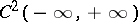and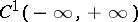, and let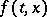be continuous together with the first derivative with respect toin the half-plane. Then the classical solution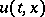in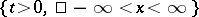of the Cauchy problem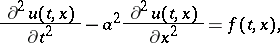(1)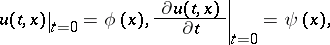(2)

is expressed by d'Alembert's formula: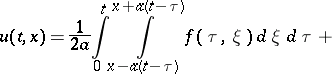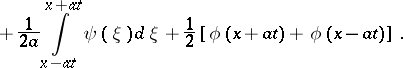If the functionsandare given and satisfy the above smoothness conditions on the interval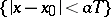, and ifsatisfies it in the triangle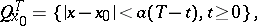then d'Alembert's formula gives the unique solution of the problem (1), (2) in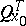. The requirements on the given functions may be weakened if one is interested in solutions in a certain generalized sense. For instance, it follows from d'Alembert's formula that ifis integrable with respect to any triangle, ifis locally integrable and ifis continuous, the weak solution of Cauchy's problem (1), (2) may be defined as a uniform limit (in any) of classical solutions with smooth data and is also expressed by d'Alembert's formula.

The formula was named after J. d'Alembert (1747).